# 555 Timer revisited

The 8-pin 555 timer is probably one of the most useful ICs ever in production, and despite its age is still being used across a multitude of applications. This article shows a few rather interesting applications, and because of it’s length will be split between the following few chapters:
1.A short Intro
2. Calculating the output parameters
3. Astable operation
4. Getting below 50% duty cycle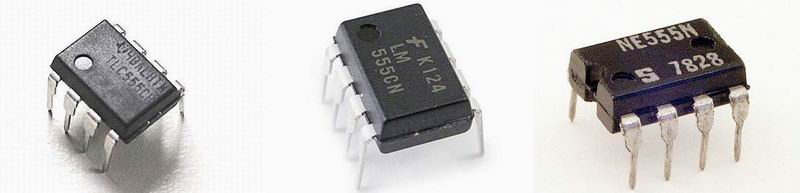### Short Intro

As I was trained in computer science rather than electronics, my first encounter with the 555 was just a few years ago, while building high voltage power supplies. The 555 was able to drive a flyback transformer, producing good voltage output but unfortunately also a lot of heat in the driver’s switching transistor:

Or another similar setup, but operating on a different frequency, for driving two induction coils in anti-parallel:

But there’s a lot more to do, and the applications are endless. Here is an ultrasonic sound generator used by a robotic “dog” to locate and follow its user, again using the 555 timer:

An electric fence perimeter protection circuit:

Or a variable duration, frequency and amplitude pulse generator circuit, used to driver a high power Hydrogen Thyratron tube:

Or a monstrous Marx Generator, pushing the limits of high voltage discharges, again using a tiny 555 to pump 12V in to 10K in the first stages of the device:

All in one, there are probably endless applications for the 555 timer. While building my high voltage inverters I recall it was somehow disappointing that the driving transistor was getting so hot so quickly, putting the entire circuit at risk. What I didn’t bother to research then, is easy to do with a little math:

### Calculating the output parameters

Usually, the regular TV flyback transformer needs to be operated on an ultrasonic frequency of 17-30KHz. By doing so, the efficiency of our high voltage inverter becomes optimum. Also the duty cycle of the PWM signal driving the switching transistor needs to be less than 50%, or our transistor will accumulate unwanted heat. Two simple facts that I often overlooked in my early constructions, will be explained here.
The 555 circuit can be adjusted for a wide variety of output signals, of various parameters, by using only a few passive components, calculated to specific values to fit the purpose.

### Astable operation

An astable circuit produces a ‘square wave’, this is a digital waveform with sharp transitions between low (0V) and high (+Vs). The circuit is called an astable because it is not stable in any state: the output is continually changing between ‘low’ and ‘high’.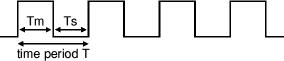The time period (T) of the square wave is the time for one complete cycle. It is easier to consider frequency (f) which is the number of cycles per second. Here is a sample circuit: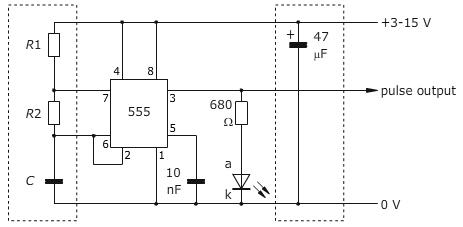By selecting values for R1, R2 and C we can determine the period/frequency and the duty cycle.
The period is the length of time it takes for the on/off cyle to repeat itself, whilst the duty cycle is the percentage of time the output is on.
In this type of circuit, the duty cycle can never be 50% or lower.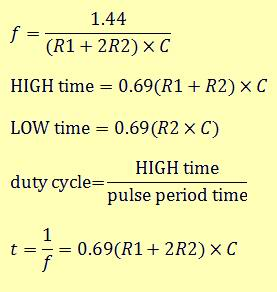To make things easier, you can use this 555 calculator script.

### Getting below 50% duty cycle

For driving a flyback transformer, we could use a capacitor of C = 1nF , a fixed R2 = 10KO and for R1 a pot of 100KO . Adjusting R1, we get a frequency interval of 12kHz .. 68kHz , enough to hit the flybacks optimum operating value, however the duty cycle is between 52% and 91%, resulting in severe transistor heating. Not the best scenario for our high voltage flyback driver. What we need is to get the duty cycle below 50%, while also keeping the frequency close to 20kHz, but this is not possible when using the 555 like pictured above.
The explanation for this is pretty simple: For the duty cycle to be 50%, the capacitor would have to charge and discharge through the same resistance. The only way to accomplish that would be to omit R1 altogether, so that the capacitor charged and discharged through R2 only.

But the problem with that is that you would end up connecting pin 7 directly to Vcc. With no resistance between pin 7 and the voltage source, the current flowing through pin 7 would exceed the maximum that can be handled by the circuitry inside the 555, and the chip would be damaged.

There’s a clever way around this limitation: Place a diode across R2. This diode bypasses R2 when the capacitor is charged. That way, the capacitor charges through R1 and discharges through R2. The circuit in this case, is: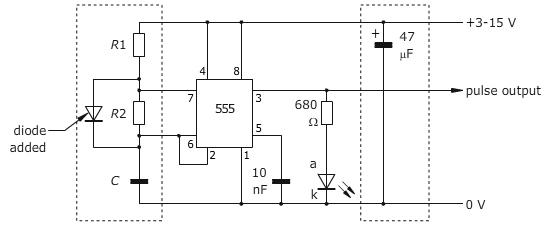When a diode is used in this way, you have complete control over the duration of both the charge and discharge time. If R1 and R2 have the same value, the capacitor takes the same amount of time to charge as it does to discharge, so the duty cycle will be 50%. If R2 is smaller than R1, the duty cycle is less than 50% because the capacitor discharges faster than it charges. The new formulas for calculating the time intervals are as follows: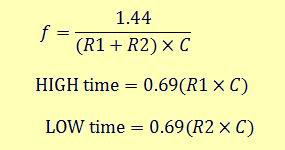To help you with the calculations, you can use the 555 diode calculator script.
All we need now, to make our flyback driver happy, is an under 50% duty cycle, 20kHz PWM signal.

To be continued.